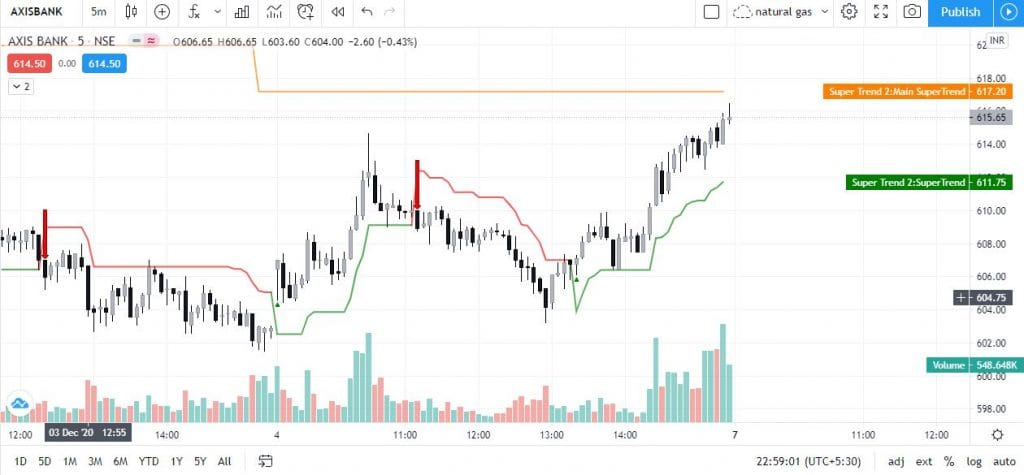# TTK INDICATOR IN FREE (SCRIPT code )

## ✅TTK INDICATOR IN FREE✔️ how to use this  ?
✔️how to apply on chart ??
✔️ how you can make some changes and .. create a tool for you ??
✔️  so for this . please watch video till End /////
✔️ and just copy this code and paste to your script editor and save as .. any name ..
✔️  and it will work flawlessly …

👇SCRIPT 👇
IF YOU COPY THEN IT WILL NOT SHOW AS YOU COPIED BUT JUST PASTE IT WILL WORK…  USE  ctrl+A  FOR COPY

t.t.k indicator in zerodha you can also use in zerodha watch this video
trend trader karan exposed you can subscribe our channel for more paid

indicators in free .. best free amibroker afl collection.

trend trader karan tool script given below simply copy and paste in pine editor
trend trader karan tool code is same thing means .. script of ttk

``````//@version=4
strategy("TTK V.2",overlay=true)
res = input(title="Resolution", type=input.resolution, defval="360",confirm = true)

ProfitPerc = input(title=" Take Profit (%)",type=input.float, minval=0.0, step=0.1, defval=1.4) * 0.01

stoploss = input(title=" Stop Loss (%)",type=input.float, minval=0.0, step=0.1, defval=0.7) * 0.01

CCI = input(20,title = "CCI")
ATR = input(5,title = "ATR")
Multiplier= 1
original = false
thisCCI = cci(close, CCI)
lastCCI = nz(thisCCI)

calcx()=>
bufferDn= high + Multiplier * wma(tr,ATR)
bufferUp= low - Multiplier * wma(tr,ATR)
if (thisCCI >= 0 and lastCCI < 0)
bufferUp := bufferDn
if (thisCCI <= 0 and lastCCI > 0)
bufferDn := bufferUp

if (thisCCI >= 0)
if (bufferUp < bufferUp)
bufferUp := bufferUp
else
if (thisCCI <= 0)
if (bufferDn > bufferDn)
bufferDn := bufferDn

x = 0.0
x := thisCCI >= 0 ?bufferUp:thisCCI <= 0 ?bufferDn:x
x

tempx = calcx()

calcswap() =>
swap = 0.0
swap := tempx>tempx?1:tempx<tempx?-1:swap
swap

tempswap = calcswap()

swap2=tempswap==1?color.yellow:color.blue
swap3=thisCCI >=0 ?color.yellow:color.blue
swap4=original?swap3:swap2

//display current timeframe's Trend

plot(tempx,color=swap4 == color.blue ? color.red : swap4,transp=0,linewidth=2)

plot(htfx,color=htfswap4,transp=0,linewidth=3)

//plotarrow( ? 1 : swap4 == color.yellow and swap4 == color.blue ? -1 :0 , title="Up Entry Arrow", colorup=color.green,colordown = color.blue, maxheight=10, minheight=10, transp=0)

plotshape( swap4 == color.blue and swap4 == color.yellow ? 1 : na , style = shape.triangleup , color = color.green , location = location.belowbar , size = size.tiny )
plotshape( swap4 == color.yellow and swap4 == color.blue ? 1 : na , style = shape.triangledown , color = color.red , location = location.abovebar , size = size.tiny)

buy =  swap4 == color.blue and swap4 == color.yellow and htfswap4 == color.yellow

sell =  swap4 == color.yellow and swap4 == color.blue and htfswap4 == color.blue

if(sell)
strategy.entry("sell",false)

if(swap4 == color.blue and swap4 == color.yellow)
strategy.close("sell")

if(swap4 == color.yellow and swap4 == color.blue )

longExitPrice  = strategy.position_avg_price * (1 + ProfitPerc)
shortExitPrice = strategy.position_avg_price * (1 - ProfitPerc)

stop_buy = strategy.position_avg_price * (1 - stoploss)
stop_sell = strategy.position_avg_price * (1 + stoploss)

if (strategy.position_size > 0)
strategy.exit(id="Target", limit=longExitPrice , stop = stop_buy)

if (strategy.position_size < 0)
strategy.exit(id="Target", limit=shortExitPrice , stop = stop_sell)
``````

### 13 thoughts on “TTK INDICATOR IN FREE (SCRIPT code )”

1.Getting this error: "line 37: 'line' could not be a variable or function name"

2.Thank you sir

3.4.5.bhai, trend magic bhi ta naa ?

6.7.I've some query. Why TTK tool accurate suggested buy and sell signal

8.hi there , one minor error buy & sell ka code nahi hai script me,
or jab manully usko add kar rahe he to error aa raha hai,

kya pura code mil sakta hai please for back testing

9.Bro….1st you need to download it in the PDF format and later copy the code from it and past it in tradingview….

10.sir is there any diff between your tool and the indian trader tool ?

11.Anonymous

Thanks for the code, if possible please provide strategy tester code also

12.lots of errors in this. could you please fix it